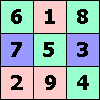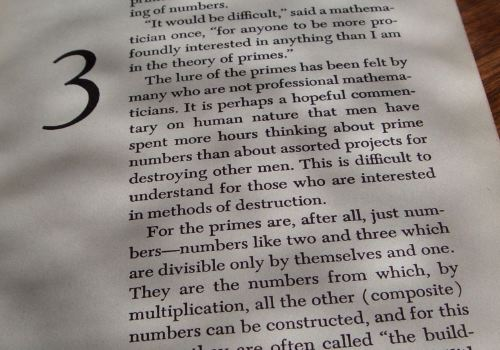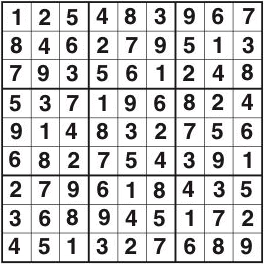ONE TO NINE by Andrew HodgesPage 3: G H Hardy's 1940 A Mathematician's Apology has remained in print, with an introduction by C. P. Snow.

Lancelot Hogben (Wikipedia).

Page 5: Constance Reid's 1956 From Zero to Infinity: What makes numbers interesting has also remained in print, with a new edition in 2006.

My comment on its place in the conformist America of the 1950s is perhaps a little unfair. One sentence in page 41, when introducing the prime numbers, makes a subtle reproof to the world of warfare.Page 7:The Times has an excellent Sudoku page and an article on its champion solver.

Page 7: The Classic Sudoku puzzle has the unique solution:Page 8: Education Guardian: 'Why doesn't anyone want to do the hard stuff any more, asks Emma Brockes - and does it matter if they don't?'

A further Guardian article asks: Does maths need a makeover?Page 16: '12 × 12 = 144' is a true statement in octal notation because 12 in octal means one eight plus two, which is ten. 144 in octal means one sixty-four plus four eights plus four, which is a hundred.

This is true for numbers to any base of 5 or more, because if n is the base,
(n + 2) × (n + 2) = n² + 4n + 4.

Nine sevens are expressed in binary notation as 1001 × 111. A long multiplication amounts to the addition of 111000 and 111. There is no carrying to be done and the result is 111111.
As a check, 111111 means 32 + 16 + 8 + 4 + 3 + 2 + 1 = 63.

The numbers with pattern 111111...111 in binary notation will make another appearance in chapter 8.

Page 17: The solution to the Killer problem is the same as to the Classic sudoku. Once you have spotted this it makes both problems very easy.

Page 21: In this sketch of the argument for the Fundamental Theorem of Arithmetic, I should perhaps have added the point that obviously 54246 cannot contain the prime factor 91457. Hence this smaller number would have two different prime factorizations.

Page 22: A government 'initiative' of 2006: Maths to become 'cool' for children in £4m campaign.

Page 31: Correction: Gödel was not from Prague, but from Brno. His adult intellectual life was based in Vienna. (My thanks to a reader for pointing this out.)